# 2nd class combinations

From how many elements you can create 4560 combinations of the second class?

n =  96

### Step-by-step explanation: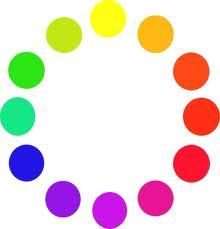Did you find an error or inaccuracy? Feel free to write us. Thank you!Tips to related online calculators
Looking for help with calculating roots of a quadratic equation?
Would you like to compute count of combinations?

## Related math problems and questions:

• CombinationsFrom how many elements we can create 990 combinations 2nd class without repeating?
• 2nd class variationsFrom how many elements you can create 2450 variations of the second class?
• CombinationsHow many elements can form six times more combinations fourth class than combination of the second class?
• CombinationsIf the number of elements increase by 3, it increases the number of combinations of the second class of these elements 5 times. How many are the elements?
• Elements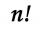If the number of elements is decreased by two the number of permutations is decreased 30 times. How many elements are?
• Two-element combinations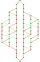Write all two-element combinations from elements a, b, c, d.
• Five-digit numbersHow many different five-digit numbers can be created from the numbers 2,3,5 if the number 2 appears in the number twice and the number 5 also twice?
• Class pairs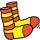In a class of 34 students, including 14 boys and 20 girls. How many couples (heterosexual, boy-girl) we can create? By what formula?
• Variations 3rd classFrom how many elements we can create 13,800 variations 3rd class without repeating?
• Committees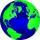How many different committees of 2 people can be formed from a class of 21 students?
• Three workplacesHow many ways can we divide nine workers into three workplaces if they need four workers in the first workplace, 3 in the second workplace, and 2 in the third?
• CombinationsHow many different combinations of two-digit number divisible by 4 arises from the digits 3, 5 and 7?
• VCP equationSolve the following equation with variations, combinations and permutations: 4 V(2,x)-3 C(2,x+ 1) - x P(2) = 0
• Two groupsThe group of 10 girls should be divided into two groups with at least 4 girls in each group. How many ways can this be done?
• How many 2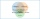How many three-lettered words can be formed from letters A B C D E G H if repeats are: a) not allowed b) allowed.
• Weekly serviceIn the class are 20 pupils. How many opportunities have the teacher selected two pupils who will have a week-class service randomly?How many ways can we thread 4 red, 5 blue, and 6 yellow beads onto a thread?